# Human in the Loop: Deep Learning without Wasteful Labelling

### Affiliations

OATML, Department of Computer Science, University of Oxford

### Published

NeurIPS 2019

TLDR: In Active Learning we use a “human in the loop” approach to data labelling, reducing the amount of data that needs to be labelled drastically, and making machine learning applicable when labelling costs would be too high otherwise. In our paper we present BatchBALD: a new practical method for choosing batches of informative points in Deep Active Learning which avoids labelling redundancies that plague existing methods. Our approach is based on information theory and expands on useful intuitions. We have also made our implementation available on GitHub at https://github.com/BlackHC/BatchBALD.

### What’s Active Learning?

Using deep learning and a large labelled dataset, we are able to obtain excellent performance on a range of important tasks. Often, however, we only have access to a large unlabelled dataset. For example, it is easy to acquire lots of stock photos, but labelling these images is time consuming and expensive. This excludes many applications from benefiting from recent advances in deep learning.

In Active Learning we only ask experts to label the most informative data points instead of labelling the whole dataset upfront. The model is then retrained using these newly acquired data points and all previously labelled data points. This process is repeated until we are happy with the accuracy of our model.

To perform Active Learning, we need to define some measure of informativeness, which is often done in the form of an acquisition function. This measure is called an “acquisition function” because the score it computes determines which data points we want to acquire. We send unlabelled data points which maximise the acquisition function to an expert and ask for labels.

### The problem is…

Usually, the informativeness of unlabelled points is assessed individually, with one popular acquisition function being BALD . However, assessing informativeness individually can lead to extreme waste because a single informative point can have lots of (near-identical) copies. This means that if we naively acquire the top-K most informative points, we might end up asking an expert to label K near-identical points!### Our contribution

In our work, we efficiently expand the notion of acquisition functions to batches (sets) of data points and develop a new acquisition function that takes into account similarities between data points when acquiring a batch. For this, we take the commonly-used BALD acquisition function and extend it to BatchBALD in a grounded way, which we will explain below.

However, knowing how to score batches of points is not sufficient! We still have the challenge of finding the batch with the highest score. The naive solution would be to try all subsets of data points, but that wouldn’t work because there are exponentially many possibilities.

For our acquisition function, we found that it satisfies a very useful property called submodularity which allows us to follow a greedy approach: selecting points one by one, and conditioning each new point on all points previously added to the batch. Using the submodularity property, we can show that this greedy approach finds a subset that is “good enough” (i.e. $1-1/e$-approximate).

Overall, this leads our acquisition function BatchBALD to outperform BALD: it needs fewer iterations and fewer data points to reach high accuracy for similar batch sizes, significantly reducing redundant model retraining and expert labelling, hence cost and time.

Moreover, it is empirically as good as, but much faster than, the optimal choice of acquiring individual points sequentially, where we retrain the model after every single point acquisition.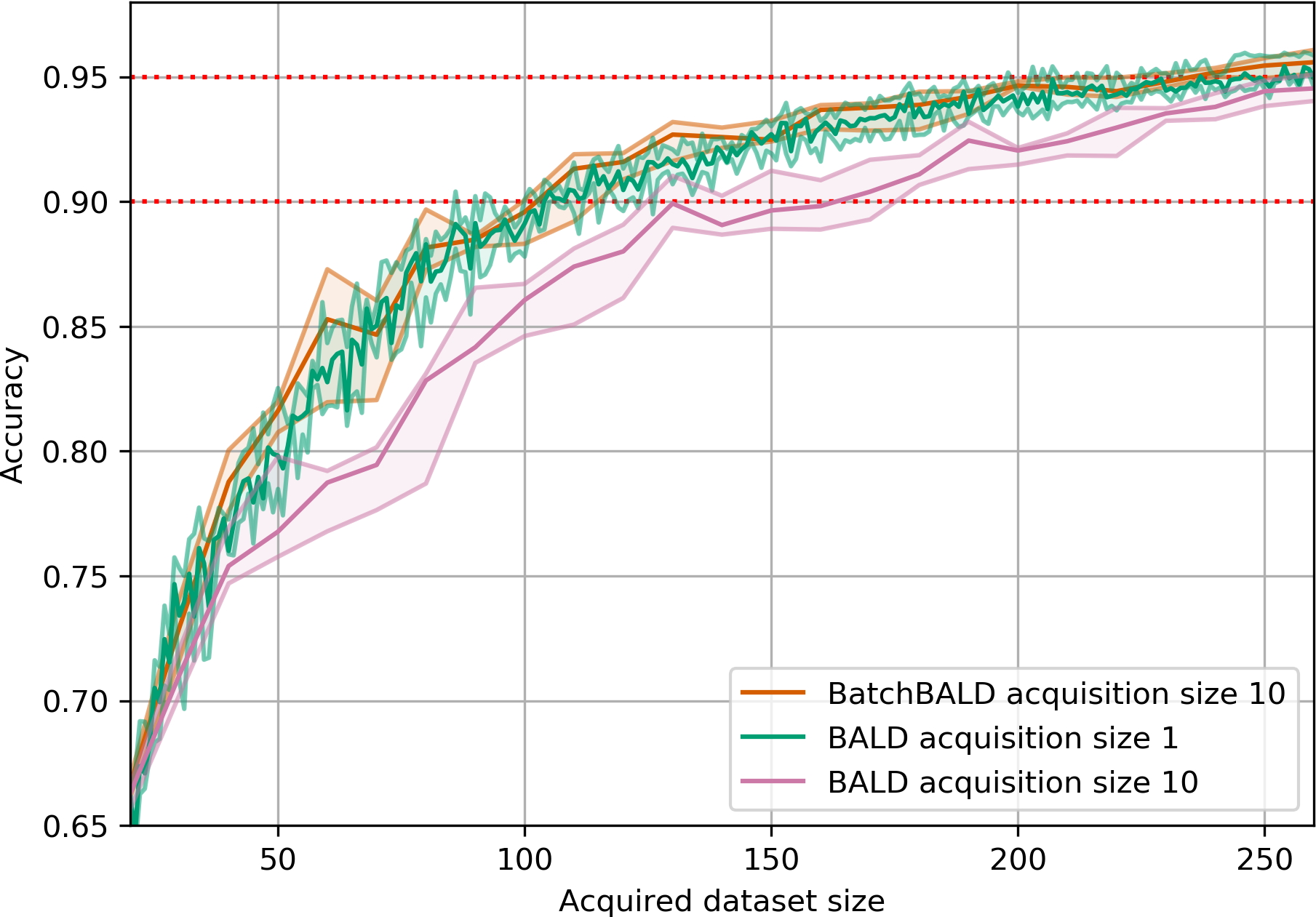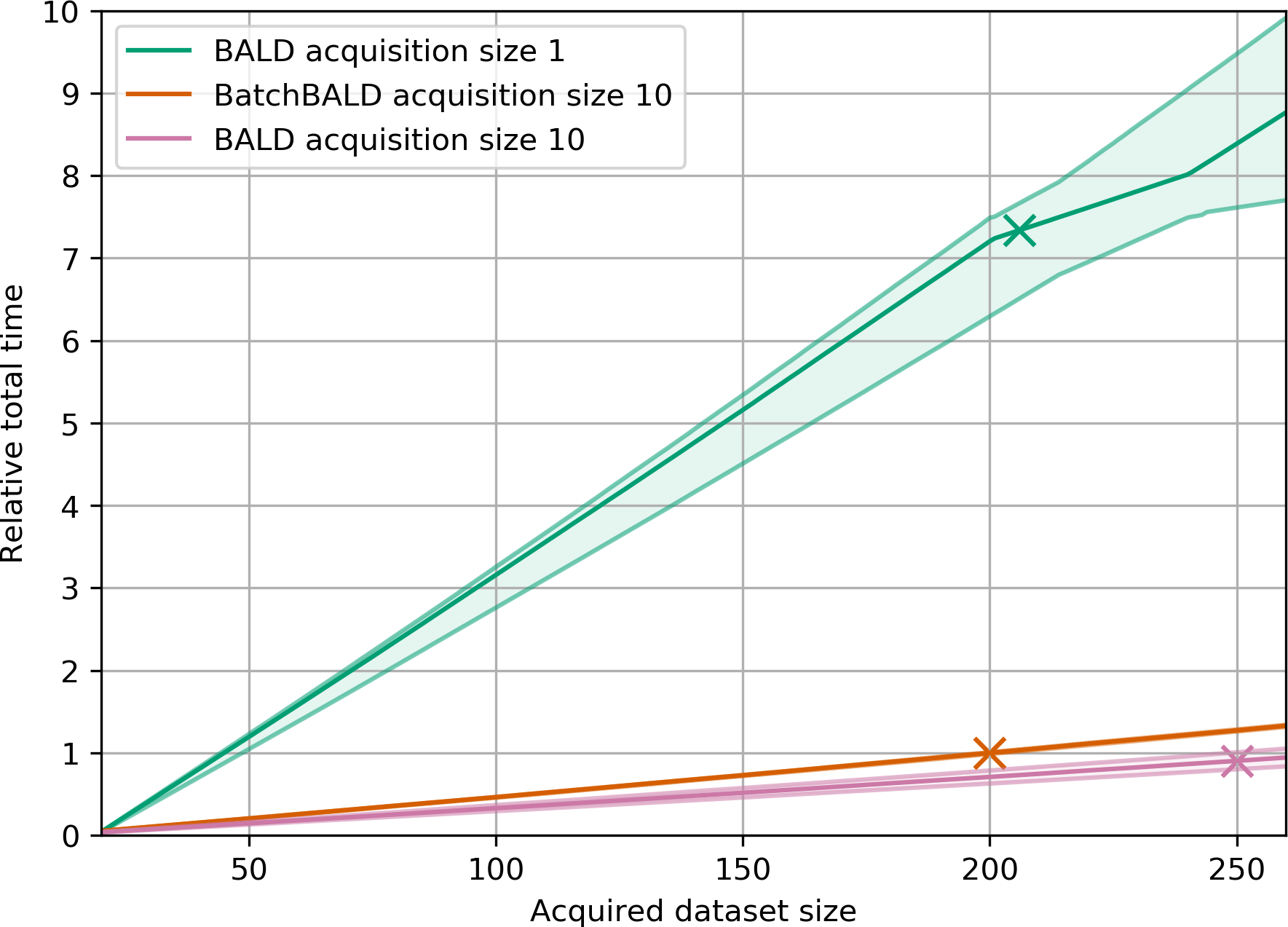Before we explain our acquisition function, however, we need to understand what the BALD acquisition function does.

### What’s BALD?

BALD stands for “Bayesian Active Learning by Disagreement” .

As the “Bayesian” in the name tells us, this assumes a Bayesian setting which allows us to capture uncertainties in the predictions of our model. In a Bayesian model, the parameters are not just numbers (point estimates) that get updated during training but probability distributions.

This allows the model to quantify its beliefs: a wide distributions for a parameter means that the model is uncertain about its true value, whereas a narrow one quantifies high certainty.

BALD scores a data point $x$ based on how well the model’s predictions $y$ inform us about the model parameters $\boldsymbol{\omega}$. For this, it computes the mutual information $\mathbb{I}(y, \boldsymbol{\omega})$. Mutual information is well-known in information theory and captures the information overlap between quantities.

When using the BALD acquisition function to select a batch of $b$ points, we select the top-$b$ points with highest BALD scores, which is standard practice in the field. This is the same as maximising the following batch acquisition function $a_{\mathrm{BALD}}\left(\left\{x_{1}, \ldots, x_{b}\right\}, \mathrm{p}\left(\boldsymbol{\omega} | \mathcal{D}_{\mathrm{train}}\right)\right) :=\sum_{i=1}^{b} \mathbb{I}\left(y_{i} ; \boldsymbol{\omega} | x_{i}, \mathcal{D}_{\mathrm{train}}\right)$ with $\left\{x_{1}^{*}, \ldots, x_{b}^{*}\right\} := \underset{\left\{x_{1}, \ldots, x_{b}\right\} \subseteq \mathcal{D}_{\text { pool }}}{\arg \max } a_{\mathrm{BALD}}\left(\left\{\boldsymbol{x}_{1}, \ldots, \boldsymbol{x}_{b}\right\}, \mathrm{p}\left(\boldsymbol{\omega} | \mathcal{D}_{\text { train }}\right)\right).$ Intuitively, if we imagine the information content of the predictions given some data points and the model parameters as sets in the batch case, the mutual information can be seen as intersection of these sets, which captures the notion that mutual information measures the information overlap.

In fact, Yeung shows that this intuition is well-grounded, and we can define an information measure $\mu^*$ that allows us to express information-theoretic quantities using set operations: \begin{aligned} \mathbb{H}(x,y) &= \mu^*(x \cup y) \\ \mathbb{I}(x,y) &= \mu^*(x \cap y) \\ \mathbb{E}_{p(y)} \mathbb{H}(x | y) &= \mu^*(x \setminus y) \end{aligned} Figure 5 visualizes the scores that BALD computes as area of the intersection of these sets when acquiring a batch of 3 points. Because BALD is a simple sum, mutual information between data points is double-counted, and BALD overestimates the true mutual information. This is why naively using BALD in a dataset with lots of (near-identical) copies of the same point will lead us to select all the copies: we double count the mutual information intersection between all!

### BatchBALD

In order to avoid double-counting, we want to compute the quantity $\mu^*(\bigcup_i y_i \cap \boldsymbol{\omega})$  , as depicted in figure 6, which corresponds to the mutual information $\mathbb{I}(y_1,…,y_b ; \boldsymbol{\omega} | x_1,…,x_b, \mathcal{D}_\mathrm{train})$ between the joint of the $y_i$ and $\boldsymbol{\omega}$  : $a_{\mathrm{BatchBALD}}\left(\left\{x_{1}, \ldots, x_{b}\right\}, \mathrm{p}\left(\boldsymbol{\omega} | \mathcal{D}_{\mathrm{train}}\right)\right) := \mathbb{I}\left(y_{1}, \ldots, y_{b} ; \boldsymbol{\omega} | x_{1}, \ldots, x_{b}, \mathcal{D}_{\mathrm{train}}\right).$ Expanding the definition of the mutual information, we obtain the difference between the following two terms: $a_{\mathrm{BatchBALD}}\left(\left\{x_{1}, \ldots, x_{b}\right\}, \mathrm{p}(\boldsymbol{\omega} | \mathcal{D}_{\mathrm{train}})\right) = \mathbb{H}\left(y_{1}, \ldots, y_{b}\right | x_{1}, \ldots, x_{b}, \mathcal{D}_{\mathrm{train}})-\mathbb{E}_{\mathrm{p}(\boldsymbol{\omega} | \mathcal{D}_{\mathrm{train}} )}\left[\mathbb{H}\left(y_{1}, \ldots, y_{b} | x_{1}, \ldots, x_{b}, \boldsymbol{\omega}\right)\right].$ The first term captures the general uncertainty of the model. The second term captures the expected uncertainty for a given draw of the model parameters.

We can see that the score is going to be large when the model has different explanations for the data point that it is confident about individually (yielding a small second term) but the predictions are disagreeing with each other (yielding a large first term), hence the “by Disagreement” in the name.

### Submodularity

Now to determine which data points to acquire, we are going to use submodularity.

Submodularity tells us that there are diminishing returns: selecting two points increases the score more than just adding either one of them individually but less than the separate improvements together:
Given a function $f: \Omega \to \mathbb{R}$, we call $f$ submodular, if: $f(A \cup \{x, y\}) - f(A) \le \left ( f(A \cup \{x\}) - f(A) \right ) + \left ( f(A \cup \{y\}) - f(A) \right ),$ for all $A \subseteq \Omega$ and elements $x,y \in \Omega$.
We show in appendix A of the paper that our acquisition function fulfils this property.

Nemhauser et al. have shown that, for submodular functions, one can use a greedy algorithm to pick points with a guarantee that their score is at least $1-1/e \approx 63\%$ as good as the optimal one. Such an algorithm is called $1-1/e$-approximate.

The greedy algorithm starts with an empty batch $A = \{\}$ and computes $a_{\mathrm{BatchBALD}}(A \cup \{x\})$ for all unlabelled data points, adds the highest-scoring $x$ to $A$ and repeats this process until $A$ is of acquisition size.

This is explained in more detail in the paper.

### Consistent MC Dropout

We implement Bayesian neural networks using MC dropout . However, as an important difference to other implementations, we require consistent MC dropout: to be able to compute the joint entropies between data points, we need to compute $a_{\mathrm{BatchBALD}}$ using the same sampled model parameters.

To see why, we have investigated how the scores change with different sets of sampled model parameters being used in MC dropout inference in figure 7.

Without consistent MC dropout, scores would be sampled using different sets of sampled model parameters, losing function correlations between the $y_i$’s for near-by $x_i$’s, and would essentially be no different than random acquisition given the spread of their scores.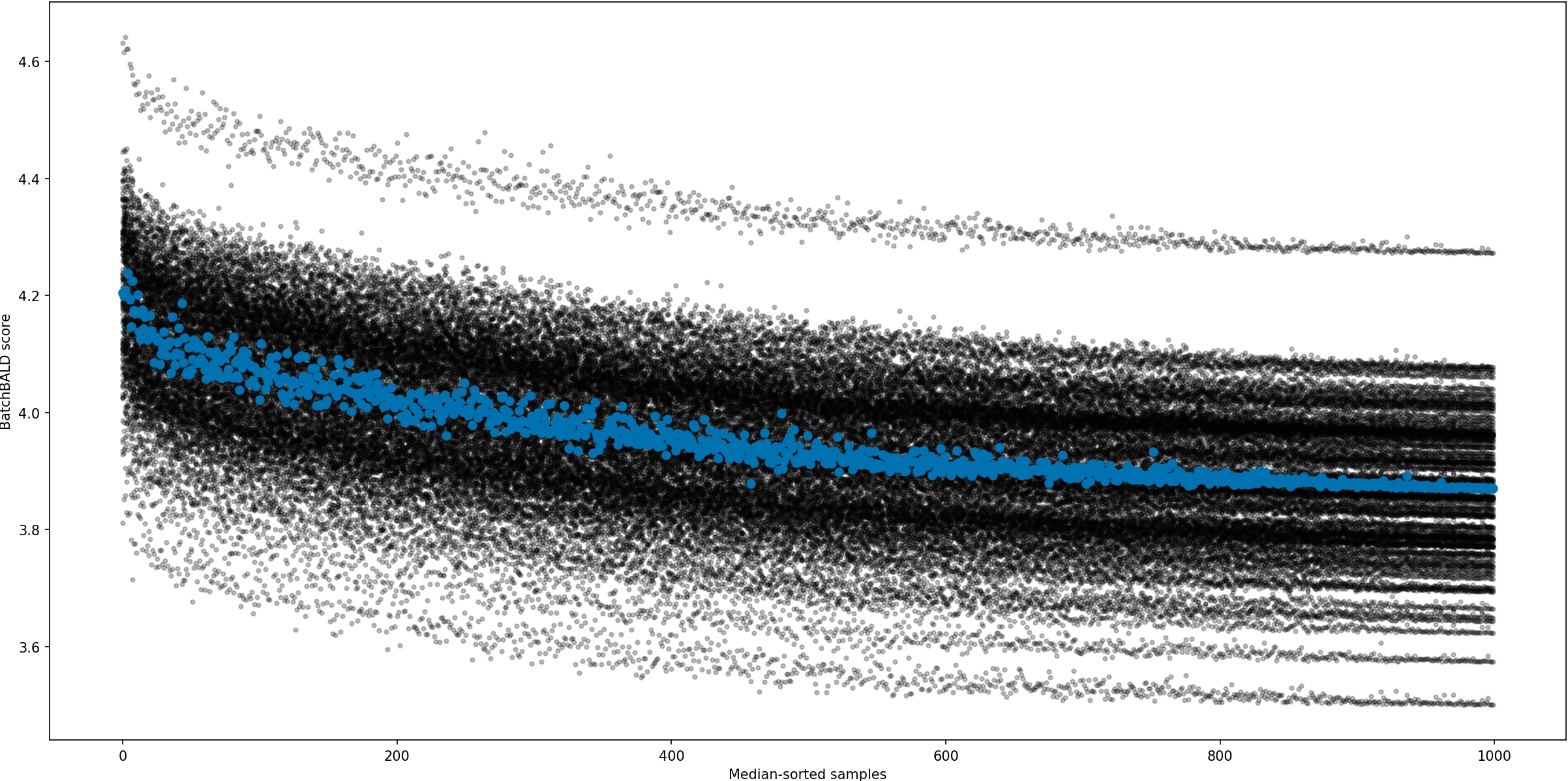### Experiments on MNIST, Repeated MNIST and EMNIST

We have run experiments on classifying EMNIST, which is a dataset of handwritten letters and digits consisting of 47 classes and 120000 data points.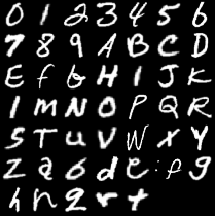We can show improvement over BALD which performs worse (even compared to random acquisition!) when acquiring large batches: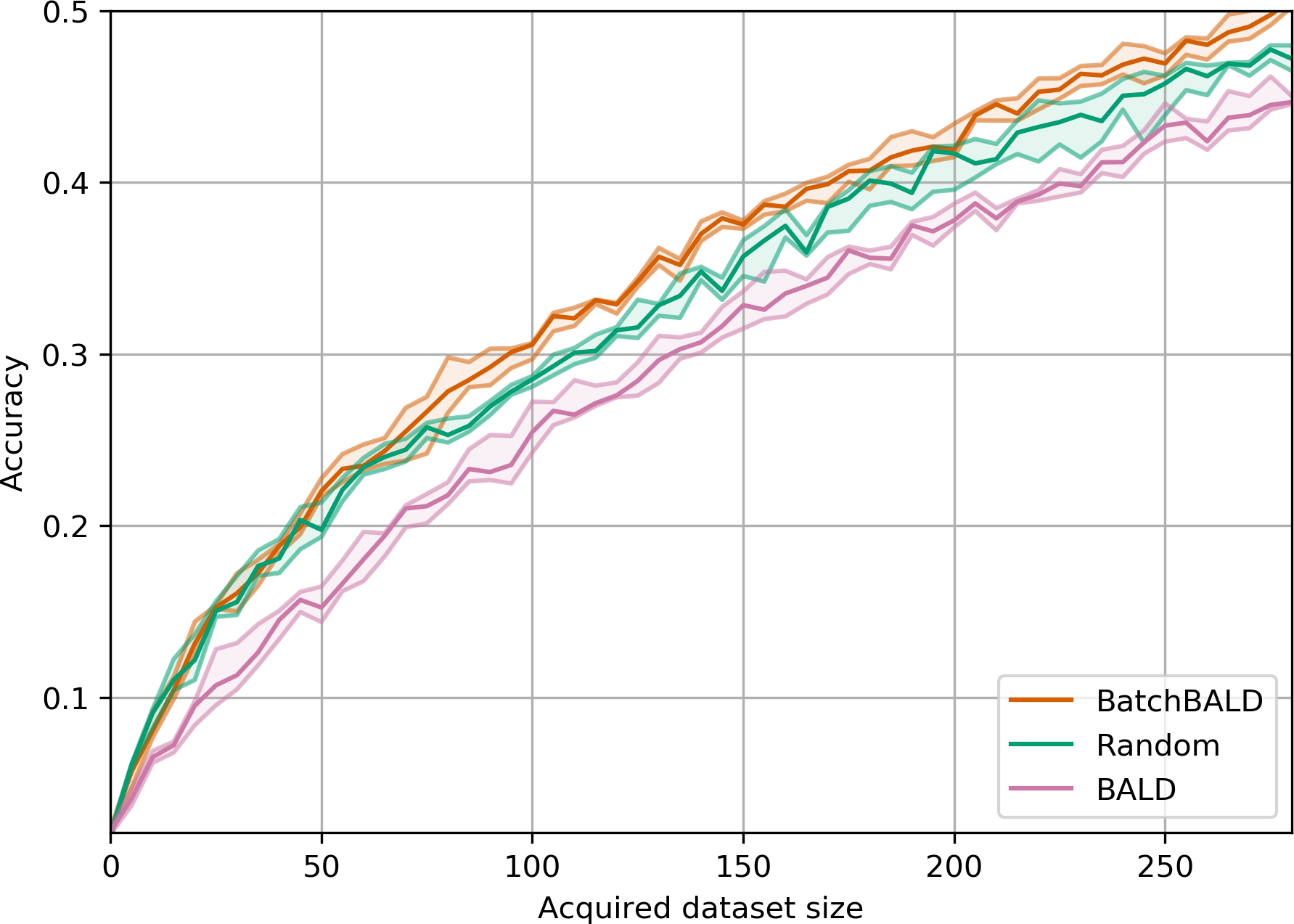This is because compared to BatchBALD and random, BALD actively selects redundant points. To understand this better, we can look at the acquired class labels and compute the entropy of their distribution. The higher the entropy, the more diverse the acquired labels are: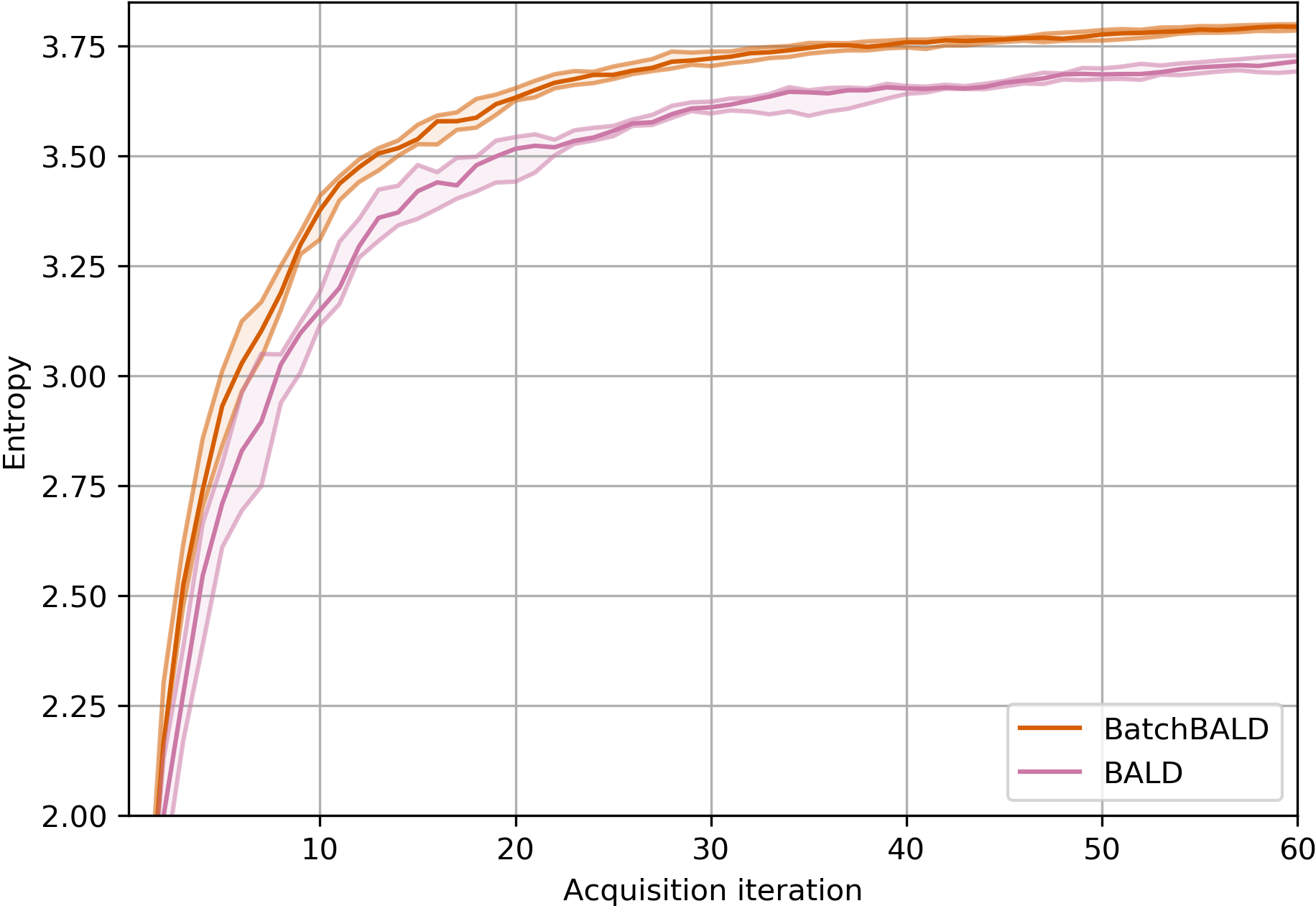We can also look at the actual distribution of acquired classes at the end of training, and see that BALD undersamples some classes while BatchBALD manages to pick data points from different classes more uniformly (without knowing the classes, of course). Random acquisition also picks classes more uniformly than BALD, but not as well as BatchBALD.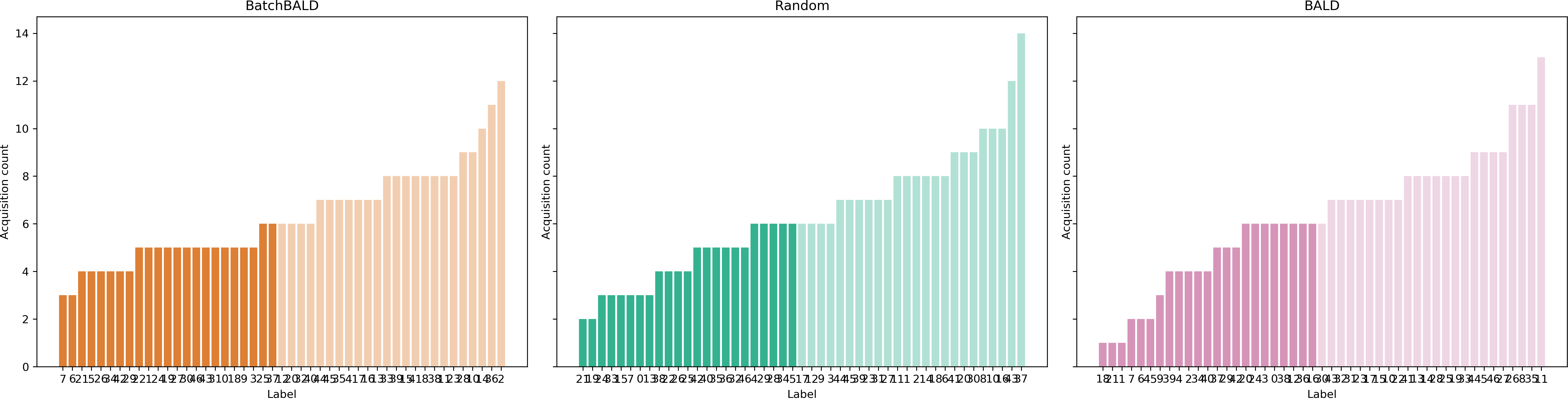Figure 14: Histogram of acquired class labels on EMNIST. BatchBALD left, random acquisition center, and BALD right. Classes are sorted by number of acquisitions. Several EMNIST classes are underrepresented in BALD and random acquisition while BatchBALD acquires classes more uniformly. The histograms were created from all acquired points.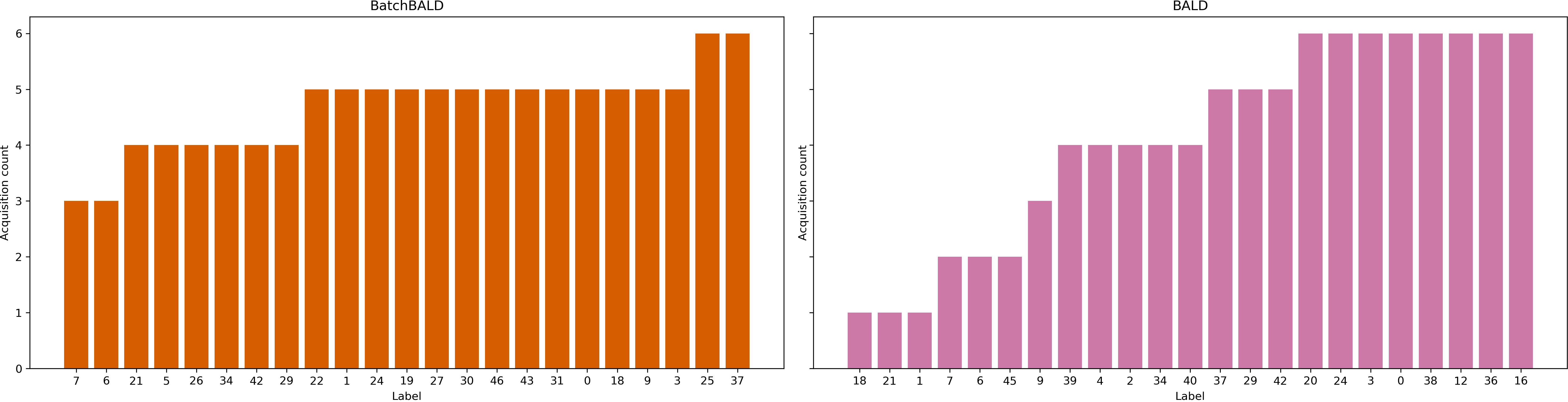To see how much better BatchBALD copes with pathological cases, we also experimented with a version of MNIST that we call Repeated MNIST. It is simply MNIST repeated 3 time with some added Gaussian noise and shows how BALD falls into a trap where picking the top $b$ individual points is detrimental because there are too many similar points. But BALD is not the only acquisition function to fail in this regime.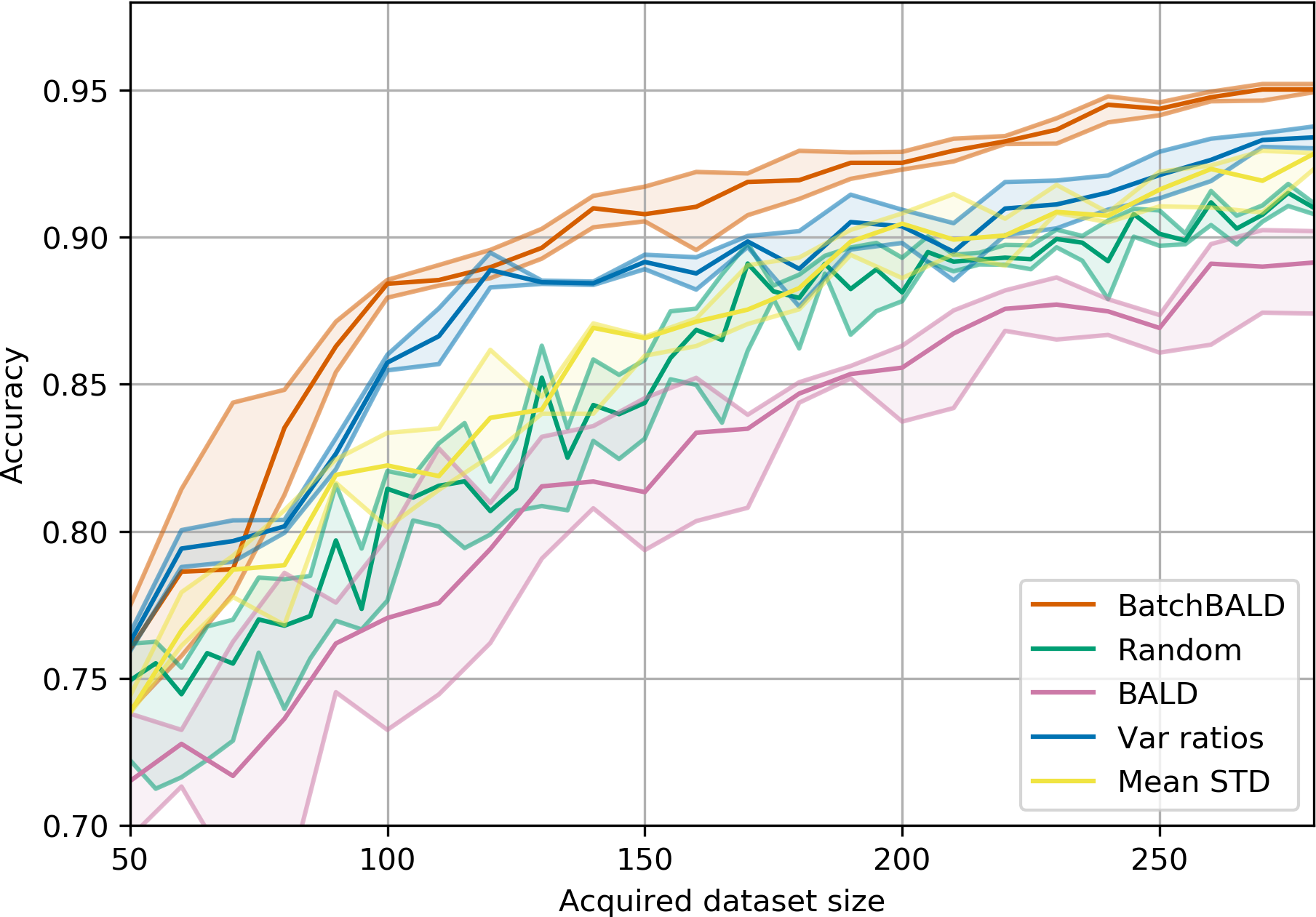Figure 15: Performance on Repeated MNIST. BALD, BatchBALD, Var Ratios, Mean STD and random acquisition: acquisition size 10 with 10 MC dropout samples.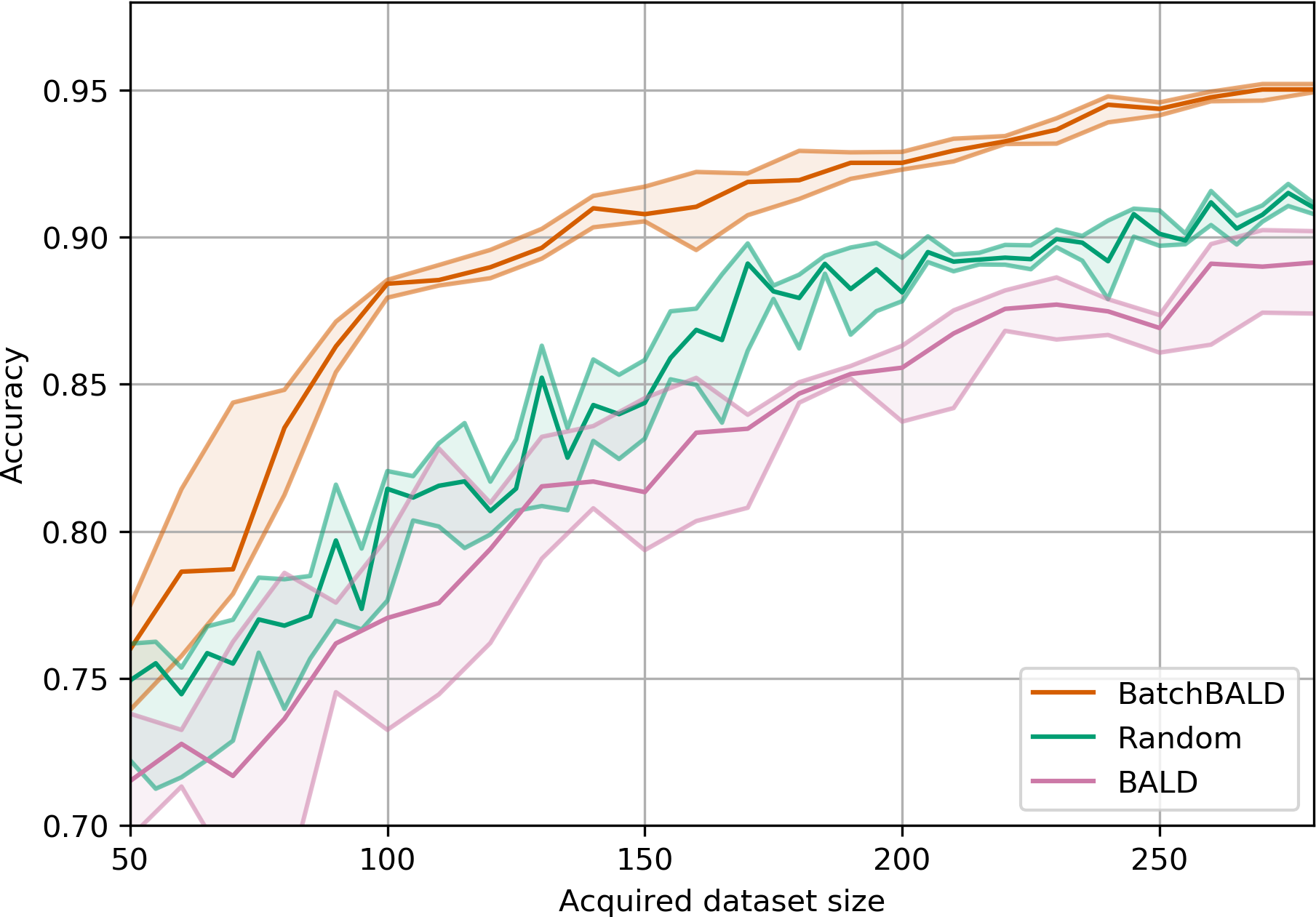We also played around with different acquisition sizes and found that on MNIST, BatchBALD can even acquire 40 points at a time with little loss of data efficiency while BALD deteriorates quickly.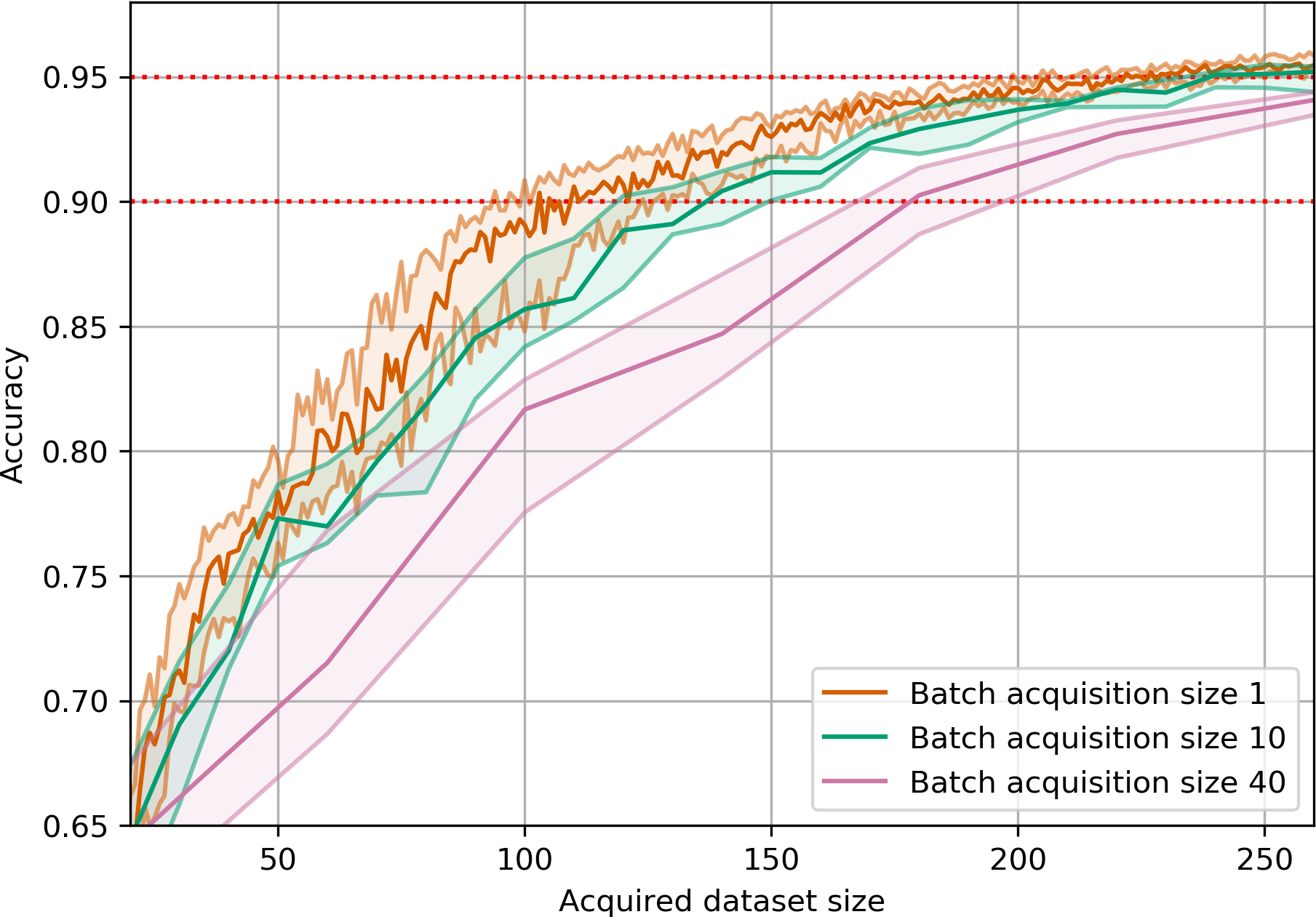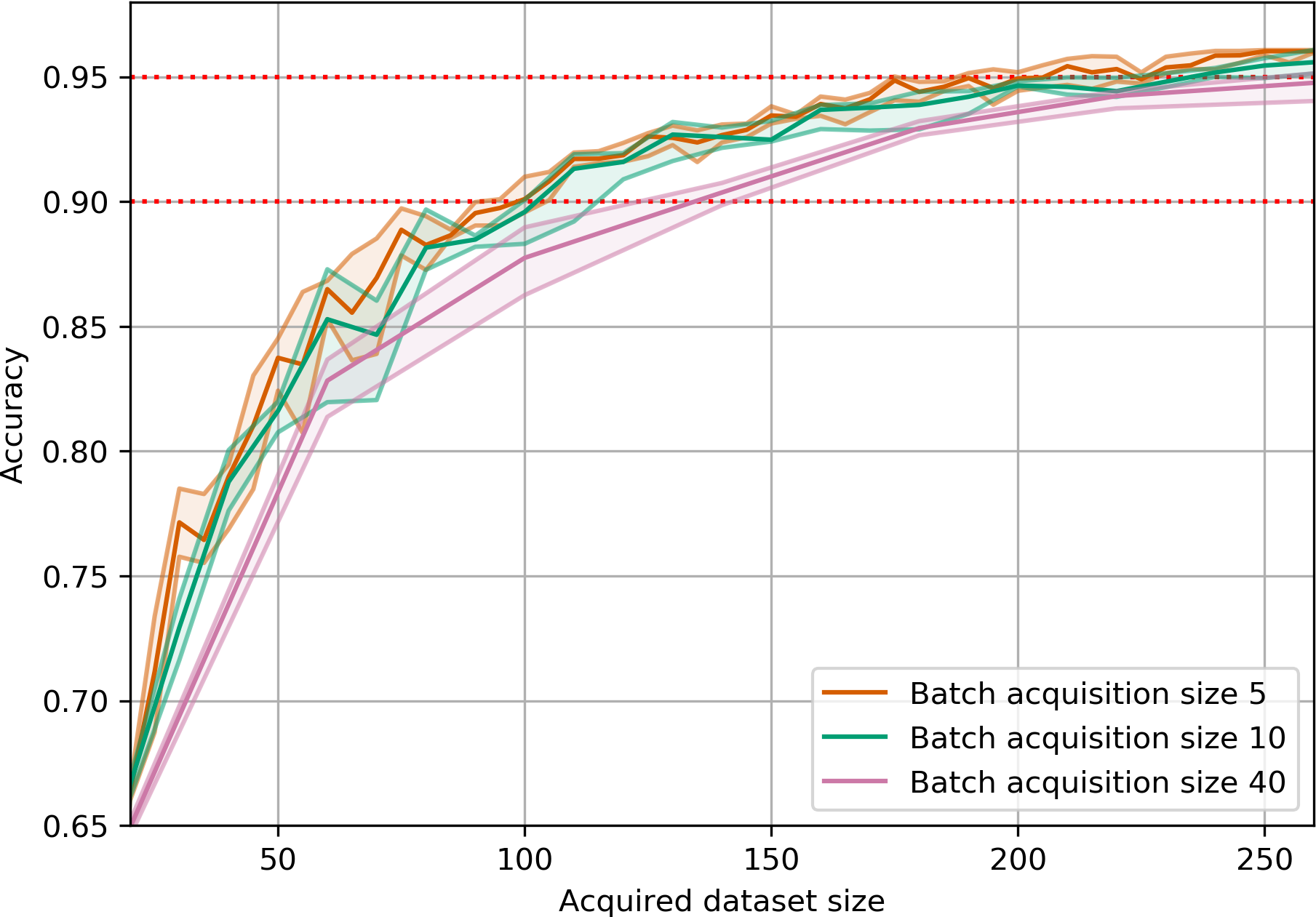### Final thoughts

We found it quite surprising that a standard acquisition function, used widely in active learning, performed worse even compared to a random baseline, when evaluated on batches of data. We enjoyed digging into the core of the problem, trying to understand why it failed, which led to some new insights about the way we use information theory tools in the field. In many ways, the true lesson here is that when something fails — pause and think.

1. BatchBALD: Efficient and Diverse Batch Acquisition for Deep Bayesian Active Learning
Kirsch, A., van Amersfoort, J. and Gal, Y., 2019.
2. Bayesian active learning for classification and preference learning
Houlsby, N., Huszar, F., Ghahramani, Z. and Lengyel, M., 2011. arXiv preprint arXiv:1112.5745.
3. A new outlook on Shannon's information measures
Yeung, R.W., 1991. IEEE transactions on information theory, Vol 37(3), pp. 466--474. IEEE.
4. An analysis of approximations for maximizing submodular set functions—I
Nemhauser, G.L., Wolsey, L.A. and Fisher, M.L., 1978. Mathematical programming, Vol 14(1), pp. 265--294. Springer.
5. Dropout as a Bayesian approximation: Representing model uncertainty in deep learning
Gal, Y. and Ghahramani, Z., 2016. international conference on machine learning, pp. 1050--1059.

## Contact

We are located at
Department of Computer Science, University of Oxford
Wolfson Building